# 10. The Discrete Fourier Transform¶

In :
import numpy as np
import matplotlib.pyplot as plt
%matplotlib inline
plt.rcParams.update({'font.size': 14})


## Q1. Finding the needle in the haystack¶

In :
# Load the first data set and plot.
plt.plot(ts, xs, '-'); plt.xlabel('t'); plt.ylabel('x(t)');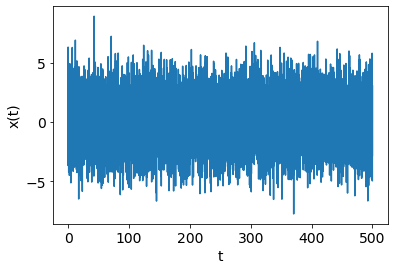In :
# Take the Fourier transform:
X_tilde = np.fft.fft(xs)
n = len(xs) # Number of data points
dt = ts-ts # Delta t, the time interval
dw = 2*np.pi/dt # Delta omega, the frequency interval
ws = np.fft.fftfreq(n, d=1/dw) # Get the frequency values, for x-axis.
plt.xlim(0, 20)
# Plot the square magnitude of Fourier coefficients
plt.plot(ws, X_tilde.real**2 + X_tilde.imag**2, '-')
plt.xlabel("angular frequency $\omega$");
plt.ylabel("$|\\tilde{X}_k|^2$");
plt.title("The Fourier transform of data file 1")
# The three spikes show evidence for a hidden periodic signal in the data.

Out:
Text(0.5, 1.0, 'The Fourier transform of data file 1')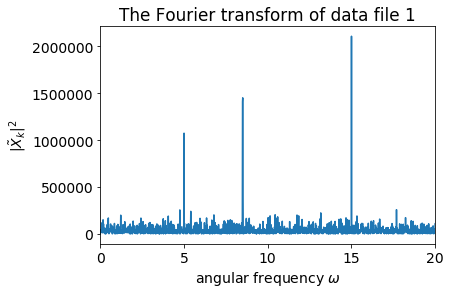## Q2. Filtering¶

In :
# Load the second data set and plot.
plt.plot(ts, xs, '-'); plt.xlabel('t'); plt.ylabel('x(t)');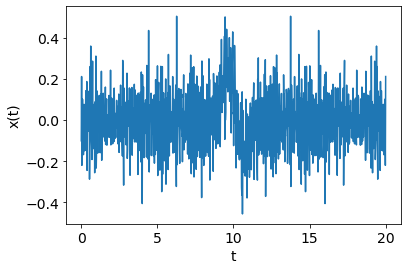In :
# Take the Fourier transform:
X_tilde = np.fft.fft(xs)
n = len(xs) # Number of data points
dt = ts-ts # Delta t, the time interval
dw = 2*np.pi/dt # Delta omega, the frequency interval
ws = np.fft.fftfreq(n, d=1/dw) # Get the frequency values, for x-axis.
# Plot the square magnitude of Fourier coefficients
plt.plot(ws, X_tilde.real**2 + X_tilde.imag**2, '-')
plt.xlabel("angular frequency $\omega$");
plt.ylabel("$|\\tilde{X}_k|^2$");
plt.title("The Fourier transform of data file 2")
# There is a regular feature in the middle, at low frequency, and irregular 'noise' at high frequencies.
# Now I will apply a filter to remove the high frequency noise.

Out:
Text(0.5, 1.0, 'The Fourier transform of data file 2')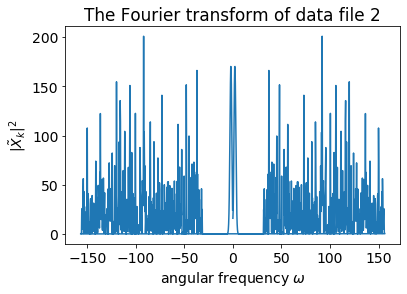In :
# Below is a crude-but-effective way to apply the filter in this case - but see the lecture notes for the dangers of apply sharp filter (Gibbs phenomenon).
omegamax = 20.0
for i in range(len(X_tilde)):
if np.abs(ws[i]) > omegamax:
X_tilde[i] = 0.0

plt.plot(ws, np.abs(X_tilde)**2, '-')
plt.xlabel("angular frequency $\omega$");
plt.ylabel("$|\\tilde{X}_k|^2$");
plt.title("After filtering in the frequency domain")
# Note that the high-frequency components have been removed.

Out:
Text(0.5, 1.0, 'After filtering in the frequency domain')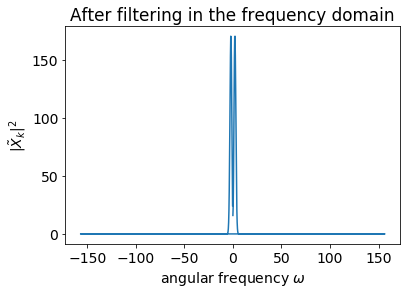In :
# Now apply the inverse Fourier transform to the filtered Fourier coefficients return to the time domain.
xs2 = np.fft.ifft(X_tilde).real
plt.plot(ts, xs2, '-')
plt.xlabel("$t$")
plt.ylabel("$x(t)$")
plt.title("After removing the high-frequency noise");
# The filter has successfully removed the high frequency noise to reveal a low-frequency signal.
# Compare and contrast with the first plot in Q2.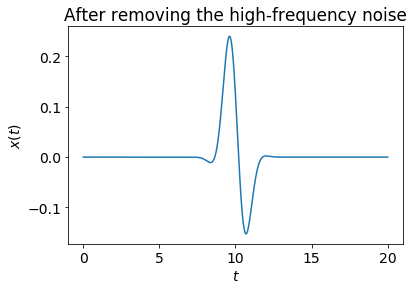## Q3. Parseval's theorem¶

In :
# Check Parseval's theorem for the first data set.
Xtilde = np.fft.fft(xs)

n = len(xs)
sum1 = (xs**2).sum()
sum2 = (np.abs(Xtilde)**2).sum() / n
print("First data set :")
print("Left-hand side : %.15e" % sum1)
print("Right-hand side: %.15e" % sum2)
print("Difference     : %.15e" % (sum1 - sum2))

First data set :
Left-hand side : 4.124038420065297e+04
Right-hand side: 4.124038420065297e+04
Difference     : 0.000000000000000e+00

In :
# Check Parseval's theorem for the second data set.
Xtilde = np.fft.fft(xs)

n = len(xs)
sum1 = (xs**2).sum()
sum2 = (np.abs(Xtilde)**2).sum() / n
print("Second data set:")
print("Left-hand side : %.15e" % sum1)
print("Right-hand side: %.15e" % sum2)
print("Difference     : %.15e" % (sum1 - sum2))

Second data set:
Left-hand side : 2.175637978052239e+01
Right-hand side: 2.175637978052239e+01
Difference     : 3.552713678800501e-15

In [ ]: